Signal Processing > Z Transform PairsShowing results 1 to 10 of 20, on page 1 of 3
 DESCRIPTION EQUATION Z-transform involving the unit step function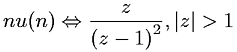Z-transform involving the unit step functionZ-transform involving the unit step function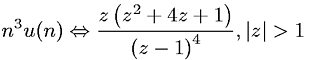Z-transform involving the unit step function and an exponentialZ-transform involving the unit step function and an exponential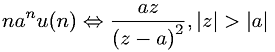Z-transform involving the unit step function and an exponentialZ-transform involving the unit step function and an exponential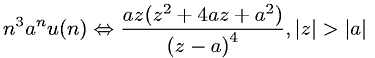Z-transform involving the unit step function and an exponential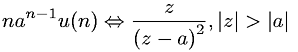Z-transform involving the unit step function and an exponential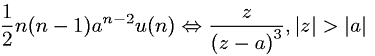Z-transform involving the unit step function and an exponential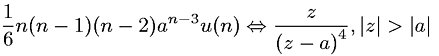Go to page: 1  2